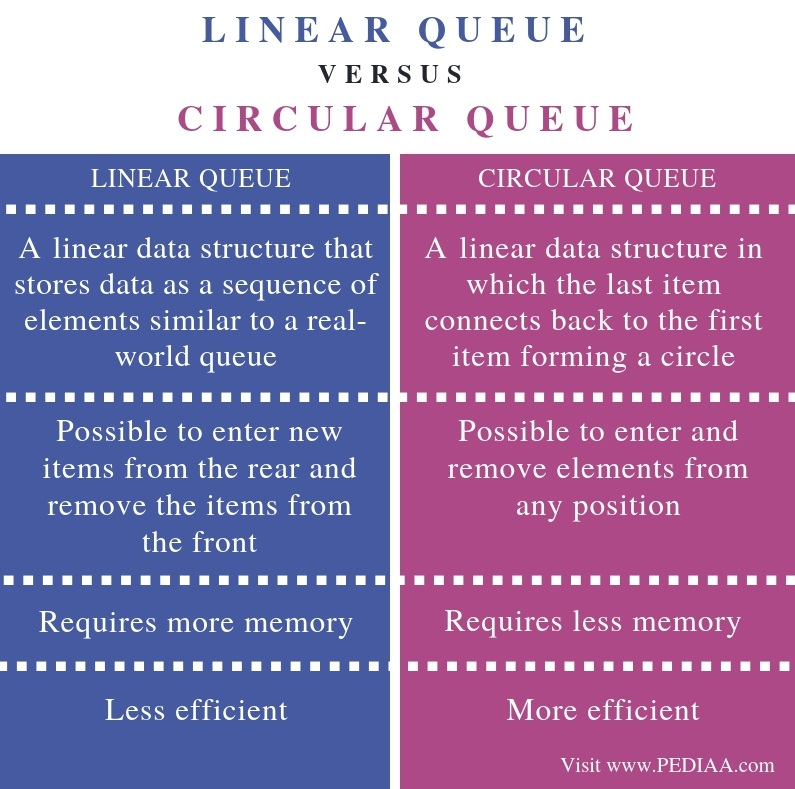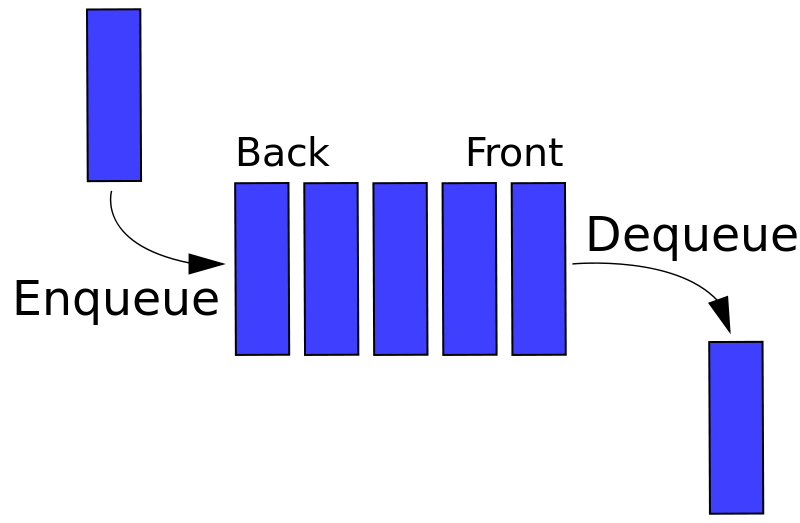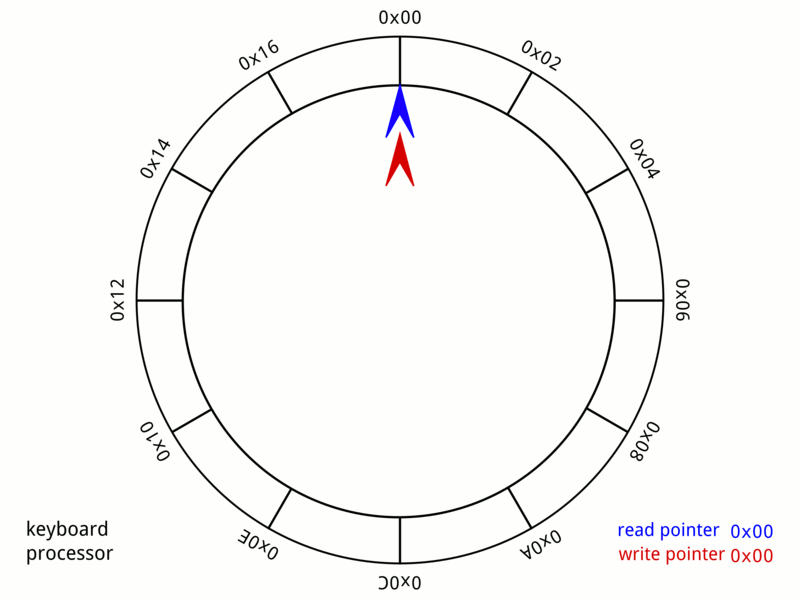# What is the Difference Between Linear Queue and Circular Queue

The main difference between linear queue and circular queue is that a linear queue arranges data in sequential order, one after the other, while a circular queue arranges data similar to a circle by connecting the last element back to the first element.

A data structure is a systematic way of organizing data to use them efficiently. It is important to consider the time and space complexity when implementing data structures. Time complexity describes the execution time while space complexity describes the memory requirement of the data structure. One major data structure in computing is a queue. There are two types of queues as linear and circular queue.

### Key Areas Covered

1. What is a Linear Queue
– Definition, Functionality
2. What is a Circular Queue
– Definition, Functionality
3. What is the Difference Between Linear Queue and Circular Queue
– Comparison of Key Differences

### Key Terms

Circle Queue, Linear Queue, Queue## What is a Linear Queue

A linear queue is a queue that is similar to a straight line. It consists of a set of data elements one after the other.  Therefore, it is possible to add new elements to the queue from one end. Hence, we call this operation enqueue. Similarly, it is possible to remove the element from the queue from the other end. And, we call this operation dequeue. The front of the queue is head and end of the queue is tail or rear. In a linear queue, it is possible to insert new items from the rear and remove the items from the front. Moreover, a queue is similar to people waiting in a straight line to access ATM machine. A new person comes and joins at the end of the queue, and the first person in the queue get access to the machine.Figure 1: Linear Queue

We can perform several operations on a linear queue. We can initialize a queue to zero. We can also check whether the queue is empty or not. Another operation is to find whether the queue is empty or not. These are some common operations to perform on a linear queue, in addition to enqueue and dequeue operations.

Even though a linear queue is simple to implement, it has some drawbacks. Removing items from the queue can create more space. However, it can still be difficult to enter new elements as this can cause an underflow condition. A circular queue helps to overcome this issue.

## What is a Circlular Queue

In a circular queue, the last item connects back to the first item to create a circle. Therefore, a circular queue is also called a ring buffer.Figure 2: A 24-byte Keyboard Circular Queue

As a circular queue connects the two ends, the first item comes after the last item. There is no overflow condition in a circular queue until the queue is actually full. Therefore, entering a new element is easy.

Furthermore, the circular queue works according to the below two conditions. The maxSize indicates the maximum number of items the queue can consist of.

rear = (rear +1 ) % maxSize;

front = (front + 1) % maxSize;

## Difference Between Linear Queue and Circular Queue

### Definition

A linear queue is a linear data structure that stores data as a sequence of elements similar to a real-world queue whereas a circular queue is a linear data structure in which the last item connects back to the first item forming a circle. Thus, this is the main difference between linear queue and circular queue.

### Insertion and Deletion

In a linear queue, it is possible to enter new items from the rear and remove the items from the front. However, in a circular queue, it is possible to enter and remove elements from any position. Hence, this is another difference between linear queue and circular queue.

### Memory Space

Furthermore, memory space is another difference between linear queue and circular queue. Linear queue requires more memory than circular queue.

### Performance

Moreover, efficiency is another difference between linear queue and circular queue. A circular queue is more efficient than a linear queue.

### Conclusion

There are two types of queues as linear and circular queue. The main difference between linear queue and circular queue is that a linear queue arranges data in a sequential order one after the other while a circular queue arranges data similar to a circle by connecting the last element back to the first element.

##### Reference:

“1. Linear Queue Tutorial.” Network Topologies (Its Types, Advantages and Disadvantages) – IncludeHelp, Available here.
2. “Circular Queue | Set 1 (Introduction and Array Implementation).” GeeksforGeeks, 24 Dec. 2018, Available here.

##### Image Courtesy:

1. “Data Queue” By Vegpuff Own work (CC BY-SA 3.0) via Commons Wikimedia
2. “Circular Buffer Animation” By MuhannadAjjan – Own work (CC BY-SA 4.0) via Commons Wikimedia### About the Author: Lithmee

Lithmee holds a Bachelor of Science degree in Computer Systems Engineering and is reading for her Master’s degree in Computer Science. She is passionate about sharing her knowldge in the areas of programming, data science, and computer systems.